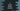# C strspn function explanation with examples# strspn() function in C with example :

strspn() function is defined in string.h header file. It is used to find out the total number of matched characters of a string in a substring. In this tutorial, I will show you how to use this method with one example program.

## Syntax of strspn() method :

The syntax of strspn() method is defined as below :

``int strspn(const char* str1, const char* str2)``

It takes two strings as the first and the second arguments. The return value is the length of the initial portion of the first string containing only the characters of the second string.

The minimum value of this function is 0 and the maximum value is the length of the second string.

### Example program :

``````#include <stdio.h>
#include<string.h>

int main()
{
char firstString;
char secondString;

printf("Enter the first string : \n");
scanf("%s",firstString);

printf("Enter the second string : \n");
scanf("%s",secondString);

int count = strspn(firstString,secondString);

printf("%d ",count);

return 0;
}``````

### Explanation :

In this program :

1. firstString and secondString are two given character array.
2. Using scanf, we are reading the user input strings to these character arrays.
3. The return value of strspn is an integer. We are storing it in variable count.
4. Finally, using printf, we are printing its value.

### Sample Output :

``````Enter the first string :
abcdef
Enter the second string :
abc``````